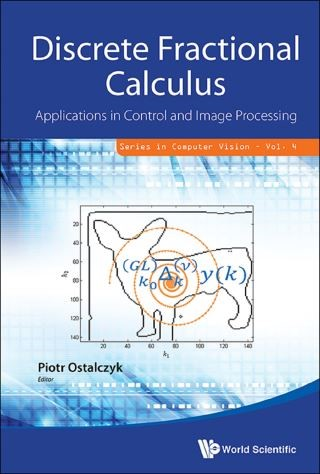## Discrete Fractional Calculus: Applications In Control And Image Processing• Description
• Author
• Info
• Reviews

### Description

The main subject of the monograph is the fractional calculus in the discrete version. The volume is divided into three main parts. Part one contains a theoretical introduction to the classical and fractional-order discrete calculus where the fundamental role is played by the backward difference and sum. In the second part, selected applications of the discrete fractional calculus in the discrete system control theory are presented. In the discrete system identification, analysis and synthesis, one can consider integer or fractional models based on the fractional-order difference equations. The third part of the book is devoted to digital image processing.

The main subject of the monograph is the fractional calculus in the discrete version. The volume is divided into three main parts. Part one contains a theoretical introduction to the classical and fractional-order discrete calculus where the fundamental role is played by the backward difference and sum. In the second part, selected applications of the discrete fractional calculus in the discrete system control theory are presented. In the discrete system identification, analysis and synthesis, one can consider integer or fractional models based on the fractional-order difference equations. The third part of the book is devoted to digital image processing.

Readership: Researchers, academics, professionals and graduate students in pattern recognition/image analysis, robotics and automated systems, systems engineering and mathematical modeling.